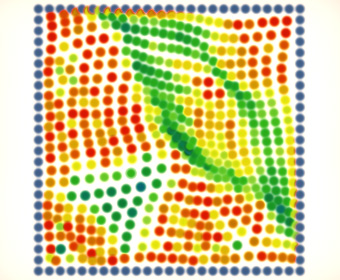## nodesThe base class `linked_node` provides functionality to create systems of interconnected turtles. It is used by two simulations: the string fixed at one end point and the net stretched on a frame. You can click and move the turtle in both simulations.
Here are some rules of using the base class to create custom nodes:

• Node must be initialized before start. Initialization has to fill the list of connected nodes: `:links`, so usually you need to create nodes and initialize them in the second pass, when all turtles are present in some structure.
At the end of initialization call `initialized :r :px :py` function. It is needed to setup the initial position of the turtle and its radius which is used to calculate a force between nodes.
• Override function `fcoeff :d` to change the default formula of the force at specified distance.
• Set `"fixed := true` to pin the turtle in its position.
• Setup a single timer to run the simulation:
``` "clock := timer [   signalw "forces   (signalw "step :dt)   signal "loss ] 20 ``` Timer interval should be short to keep high precision of step and force calculations, and long enough to let the turtles make theirs steps.
• Setup another timer to repaint the graphics window (and avoid too frequent redraws):
`"painter := timer [refresh] 50`
• There are also a few parameters like the node mass, gravity force, etc, which are easy to understand from the code. Have fun!